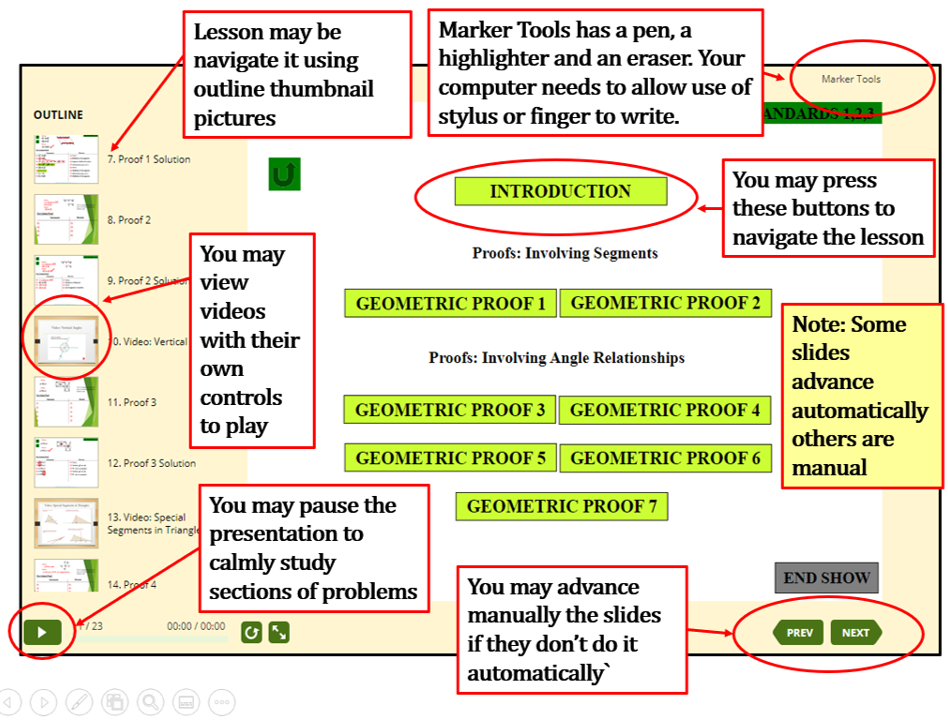QUICK SITE TOUR VIDEO OF HOW TO INTERACT

WITH THESE LESSONS (Click inside the graphic and Press Play Arrow Buttom)

# PROBABILTIY AND STATISTICS

scroll down to find the lessons

 CHAPTER I PROBABILITY AND STATISTICS TOPICS 1.- Measures of Central Tendency and Dispersion: Mean, Median and Mode. Standard Deviation and Variance. 2.- Counting Principle. 3- Combinations and Permutations. 4.- Experimental Probability (Introduction) 5.- Adding Probabilities (Module "OR") and Multiplying Probabilities (Module "AND") (From Informal to Formal) 6.- Joint, Marginal and Conditional Probability. 7- Binomial Probability. Calculated with the formula, the tables and with Ti-83 Texas Instrument Calculator. 8- Normal Distribution: Introduction to Standard Normal Distribution and Z-score 9- Central Limit Theorem Introduction: Sampling Distributions & Central Limit Theorem 10- Confidence Intervals: Standard Deviation Known and Unknown, as Well for Proportions. Linear Regression with Confidence Intervals for slope and y-intercept. 11-Hypothesis Testing Proportions 12- Hypothesis Testing Mean and Standard Deviation Known and Unknown Population Standard Deviation CHAPTER II PLAYING DICE, CARDS AND THE WHEEL OF FORTUNE. A HANDS ON APPROACH TO LEARN PROBABILITY. 1.- Real World Probability with a Spinning Wheel. (You fill in the tables) 2.- Real World Probability with a Spinning Wheel (Tables already fill) 3.- Real World Probability with Dice and Cards (You fill the tables) 3.- Real World Probability with Dice and Cards (Tables already fill) Inside Each Lesson You May Do As The Graphic IndicatesHOME

LONG DURATION DEMO OF HOW TO INTERACT (Several Minutes but worthwhile)